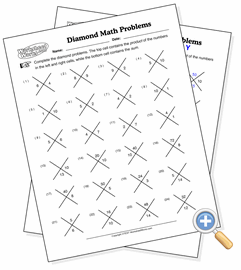# Diamond ProblemsDevelop polynomial factoring skills by reversing addition and multiplication

When students learn how to factor a polynomial such as x2 - 8x + 15, one of the skills they need to develop is to find two numbers which can be added to get one number and multiplied to get another. A diamond problem is designed to focus on just this skill. The left and right numbers in the problem are the factors. The top number is for the product, and the bottom is for the sum.

One way to introduce this skill to students is to have three forms of diamond problems on the page and make them increasingly difficult. The first form is simply having bot factors present, in which case it’s simple addition and multiplication. The second removes one factor, but provides either the sum or the product. At the bottom of the page, the problems only provide the sums and products, and the student is forced to derive both factors by finding the right pair which make up both.